• EXCEL和VBA里默认的数值一般是int 尤其是VBAint是很不够用的 int的数值范围很小，只支持 65535 ，稍微大一点的计算，可能就报错了，会显示 “数值溢出” 处理方法 clng(500000) randmize clng(1+(500000-1...

EXCEL和VBA里默认的数值一般是int

尤其是VBA里int是很不够用的

int的数值范围很小，只支持 65535 ，稍微大一点的计算，可能就报错了，会显示 “数值溢出”

处理方法

clng(500000)

randmize

clng(1+(500000-1)*RND)

展开全文vba
• VBA函数：int（）函数

千次阅读 2020-08-25 19:55:05
VBA函数：int（）函数 int(x)函数是取不大于x的最大整数，这个函数用法如下： 'int(x)函数是取不大于x的最大整数，这个函数用法如下： Sub int_fun() Debug.Print Int(4.88) Debug.Print Int(0.88) Debug....

VBA函数：int（）函数

int(x)函数是取不大于x的最大整数，这个函数用法如下：

'int(x)函数是取不大于x的最大整数，这个函数用法如下：
Sub int_fun()
Debug.Print Int(4.88)
Debug.Print Int(0.88)
Debug.Print Int(1.88)
Debug.Print Int(-4.88)
Debug.Print Int(-0.88)
End Sub

运行结果如下所示：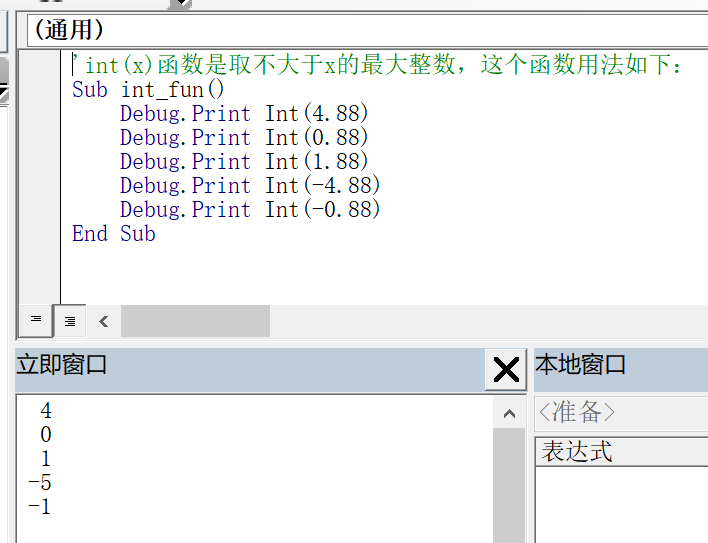展开全文• p1 = Int(1 + 10* Rnd()) ---这里正确，刚好10=10-1+1 p1 = Int(1 + (10-1+1) * Rnd()) --总是正确 如果生成 2-9之间的随机数 p2 = Int( 2 + 9* Rnd() ) --错误的 p2 = Int(2 + (9-2+1) * Rnd()) ----正确 ...

1用EXCEL生成随机数

• rand()  是 [0,1）,随机到的是小数
• randbetween(a,b)   是  [a,b] ,但是只能是整数

如果想在EXCEL公式用randbetween()随机小数呢？

• 随机，randbetween() 只能随机整数，如果是要小数，那就先乘100，随机完之后再除100这样处理
• randbetween(a,b)   是  [a,b]
• RANDBETWEEN(1000*G49,1000*H49)/1000

2 用VBA生成随机数

在VBA里

• rnd是[0,1）之间随机
• 左闭右开区间

方法

• 整数[a,b]随机正确写法为 int(a+(b-a+1)*rnd())
• 小数的随机[a,b)  随机正确写法为 (a+(b-a)*rnd()  ’但是右边还是不是闭区间有瑕疵
• 这样来说，小数随机，如果要求两边是闭区间，不如用整数随机方法，把小数*1000等变大为整数，最后再除，也是一样得把。

int(1+[0,1)*(6-1+1))

= int(1+[0,1)*6)

=int(1+[0,6))

=int([1,7))

=[1,6]

0.1+[0,1)*(0.6-0.1)

=0.1+ [0,1)*0.5

=0.1+[0,0.5)

=[0.1,0.6)

官方解释

为了生成某个范围内的随机整数，可使用以下公式：
Int((upperbound - lowerbound + 1) * Rnd + lowerbound)
这里，upperbound 是随机数范围的上限，而 lowerbound 则是随机数范围的下限。
注意 若想得到重复的随机数序列，在使用具有数值参数的 Randomize 之前直接调用具有负参数值的 Rnd。使用具有同样 number 值的 Randomize 是不会得到重复的随机数序列的。

•  rnd 是左闭右开区间   [0,1)
• 如果要生成 a~b之间的随机数，一般是int(a+ (b-a+1)*rnd)
• 因为一般都是要在整数之间随机 要用 int()

2.1 例子

生成1-10的随机数

• p1 = Int(1 + 10* Rnd())              ---这里正确，刚好10=10-1+1
• p1 = Int(1 + (10-1+1) * Rnd())     --总是正确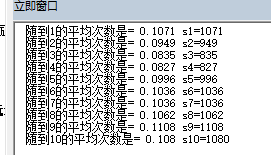如果生成 2-9之间的随机数

• p2 = Int( 2 + 9* Rnd() )              --错误的
• p2 = Int(2 + (9-2+1) * Rnd())     ----正确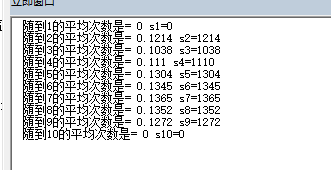2.2 还玩了下其他的

rnd(-1)

• 用永远生成相同的随机数
• 相当于固定随机数种子了？这样的应用情景是？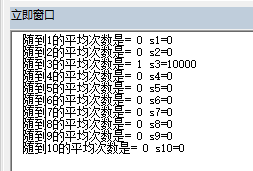rnd(0)

• 虽然随机序列都一样，但和rnd(-1) 不同
• 每次连续随机，也是一样的随机数种子，总是用上次的
• 但是如果先停止，再重新开始，就是用下一个随机种子，会和以前的不同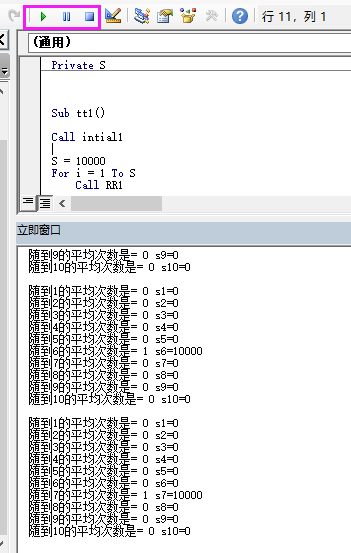3 下面是测试随机数的代码

测试方法：

• 这个进行了多轮随机
• p1 = Int(1 + (10) * Rnd())   'Rnd()分别修改为 rnd(-1)   rnd(0)  rnd(1)  rnd(999) 看效果
Private p1
Private s1, s2, s3, s4, s5, s6, s7, s8, s9, s10
Private S

Sub tt1()

Call intial1

S = 10000
For i = 1 To S
Call RR1
Call SS1
'    Debug.Print p1
Next

Call SS2
End Sub

Function intial1()

s1 = 0
s2 = 0
s3 = 0
s4 = 0
s5 = 0
s6 = 0
s7 = 0
s8 = 0
s9 = 0
s10 = 0

End Function

Function SS2()
Debug.Print "随到1的平均次数是= " & s1 / S & " s1=" & s1
Debug.Print "随到2的平均次数是= " & s2 / S & " s2=" & s2
Debug.Print "随到3的平均次数是= " & s3 / S & " s3=" & s3
Debug.Print "随到4的平均次数是= " & s4 / S & " s4=" & s4
Debug.Print "随到5的平均次数是= " & s5 / S & " s5=" & s5
Debug.Print "随到6的平均次数是= " & s6 / S & " s6=" & s6
Debug.Print "随到7的平均次数是= " & s7 / S & " s7=" & s7
Debug.Print "随到8的平均次数是= " & s8 / S & " s8=" & s8
Debug.Print "随到9的平均次数是= " & s9 / S & " s9=" & s9
Debug.Print "随到10的平均次数是= " & s10 / S & " s10=" & s10

End Function

Function RR1()

Randomize
p1 = Int(1 + (10) * Rnd())   'Rnd()分别修改为 rnd(-1)   rnd(0)  rnd(1)  rnd(999) 看效果
'Debug.Print p1
End Function

Function SS1()

Select Case p1
Case Is = 1
s1 = s1 + 1
Case Is = 2
s2 = s2 + 1
Case Is = 3
s3 = s3 + 1
Case Is = 4
s4 = s4 + 1
Case Is = 5
s5 = s5 + 1
Case Is = 6
s6 = s6 + 1
Case Is = 7
s7 = s7 + 1
Case Is = 8
s8 = s8 + 1
Case Is = 9
s9 = s9 + 1
Case Is = 10
s10 = s10 + 1
End Select

End Function

4 如果是不重复的随机数呢，之后再弄

展开全文vba
• int取整的数为负数，比如int(-1.3)，结果为2 。为什么，原因在这里http://www.vba100.com/home/coursedetial/171/1/0
展开全文excel
• VBA 数据类型相互转换 CBool(expression) CByte(expression) CCur(expression) CDate(expression) CDbl(expression) CDec(expression) CInt(expression) CLng(expression) CSng(expression...
• CStr 函数示例本示例使用 CStr 函数将一数值转换为 String。Dim MyDouble, MyStringMyDouble = 437.324 ' MyDouble 为 Double...CInt 函数示例本示例使用 CInt 函数将一数值转换为 Integer。Dim My...
• Base 类型 Byte Boolean single! currency@ Double# date ## integer% long & decimal String [*10] \$ Object null is noting Variant ... (...
• iamlaosong文 1、程序中如果只用Rnd取随机数时，每次执行都会产生相同的随机数序列。 2、Randomize可以让每次执行程序产生不同的序列，因为该语句用计时器当前值做随机序列的种子。 3、同一个程序中Randomize number...vba
• vba中数据类型转换函数

万次阅读 2014-07-02 17:05:57
CInt(expression) 转换为Integer型 CLng(expression) 转换为Long型 CSng(expression) 转换为Single型 CStr(expression) 转换为String型 CVar(expression) 转换为Variant型 Val(string) 转换为数据型 Str(number) ...vba 函数
• Excel VBA 类型转换函数

万次阅读 2010-08-11 17:17:00vba excel integer date string
• Sub 宏1() Dim M As String Dim arrResult() As String For i = 1 To 365 ... Cells(i, 2).Interior.Color = RGB(CInt(arrResult(0)), CInt(arrResult(1)), CInt(arrResult(2))) 'For i = LBound(...vba
• Abs 函数 ...Sgn 函数、数学函数中的Abs 函数、Atn 函数、Cos 函数、Exp 函数、Fix 函数、Int 函数、Log 函数、Rnd 函数、Sgn 函数、Sin 函数、Sqr 函数、Tan 函数、导出的数学函数： 导出的数学函数Excel VBA
• VBA基础语法：类型转换函数

千次阅读 2020-01-02 13:34:55
是由 VBA 指定的。可以在程序中使用它们来替换真正的值。其中大部分是可以组合的，例如 vbUpperCase + vbWide ，互斥的常数不能组合，例如 vbUnicode + vbFromUnicode 。当在不适用的 区域 使用常数 vbWide 、 ...
• 1 #include  < ...int  i)  4   {  5  stringstream s;  6  s   i;  7   return  s.str();  8  }   9 转载于:https://www.cnblogs.com/skunk/archive/2009/06/04/1496036.html
• VBA调用dll实例

2010-08-12 18:58:37
VBA调用dll是指定路径的，如果该路径下没有想调的dll怎么办，本文给出了一个解决方法
• VBA中的数据类型转换

千次阅读 2020-11-24 23:47:38
VBA提供了两套内置的数据类型转换函数，第一组包含Int、 CVDate和Str,这是早期版本，只是向后兼容而保留。第二组函数都是以字母“C”开头,编程中使用第二组。 每个转换函数的语法基本相同，例如： Expression是...
• 在Excel VBA中解决这些问题的基础是首先生成一系列随机数. 今天，我们将了解如何使用VBA生成满足我们需求的随机数. 在Excel中生成随机数的函数是Rnd，在VBA中，随机函数也是Rnd. Rnd函数生成的随机数范围是[0,1)，...
• vba 禁止用户添加sheet。。。。 禁止 新增工作表vba 开发
• TRUE，否则返回 FALSE 2.2.3为负数或者不是整数，使用int函数和Excel的RoundUp函数，int函数取整（int(3.2)=3），RoundUp向上取零个小数位（RoundUp（3.2,0）=4），如果两个值不相等就是小数 Sub test() dim ...vba excel 数据分析 数据类型
• VBA基础语法：取整函数

千次阅读 2020-01-07 21:31:03
VBA取整函数有好几个，用法也不同，有的...（1）CInt：只能在VBA中使用 CInt(12.56)=13， CInt(12.46)=12， CInt(-12.56)=-13， CInt(-12.46)=-12 （2）Round：在VBA中使用和CInt相同 Round(12.56)=13， Roun...
• 直接上代码示例： nowdate为日期型变量 strSql = “select DISTINCT 日期 from new_ubi_data ORDER BY 日期 DESC Limit 0,1” nowdate = AdoConn.Execute(strSql)(0) 在执行SQL查询语句后添加(0)即可 ...后端
• Excel VBA简单语法

万次阅读 2019-06-13 00:26:20
该文为想要学习VBA编程的会计和编程同学有一定的个性化定制Excel操作提供参考 1. VBA编程是什么 VBA 究竟是什么?更确切地讲,它是一种自动化语言,它可以使常用的程序自动化,可以创建自定义的解决方案.90 年代早期,...
• 一、vba 数据类型 1、基本数据类型 整数类型：byte、integer、long、long long 小数类型：single、double 布尔类型：boolean 字符型：string 万能类型：variant 其他类型：date、object 2、...
• std::string str1 = "abcde"...char strInt; for(int i = 0; i &lt; num; i++){ itoa(i, strInt, 10); std::string str2 = str1 + "No. " &lt;&lt; strInt; }
• Excel VBA编程

万次阅读 多人点赞 2019-04-11 16:45:51
文章目录如何创建VBAVBA语法规则声明变量给变量赋值让变量存储的数据参与运算关于声明变量的其他知识变量的作用域特殊的变量——数组声明多维数组声明动态数组其他创建数组的方法数组函数利用UBound求数组的最大索引...excel vba
• VBA实现进度条的显示 Sub Test() ' The UserForm1_Activate sub calls Main UserForm1.LabelProgress.Width = 0 UserForm1.Show End Sub Private Sub UserForm_activate() Call Main End Sub Sub Main() ' ...VBA 进度条
• '1 数据类型综述'在VBA中的数据类型有整数、文本、对象等类型。这些不同的类型有着特定的作用，在进行运算时也会占用'不同大小的内存，所以我们在编写程序时为了提高运行效率，一般都要定义数据的类型。'2 数据类型...
• VBA基础知识

千次阅读 2019-11-12 14:18:36
VBA入门学习笔记本关键词：VB、VBA、Microsoft office、excel、access、PowerPoint1.VBA的基本概念2 数据类型2.1 VBA基本数据类型2.2 VBA自定义的数据类型3 VBA常量3.1 VBA系统定义常量3.2 VBA固有常量3.2 VBA符号...EXCEL VBA
• VBA API详解

千次阅读 2020-12-17 22:27:10
Office版本变动  在office2010以前微软只提供了32-bit版本，但在2010以后出现了32-bit和64-bit两个版本...后者能处理更大的数据和VBA代码运行速度得到提升。代码的兼容性也变得复杂，特别是API的声明。 版本区别 ...编程语言
• 各位大神，我是VBA入门级菜鸟，我就是想简单的从913条数据中随机选取其中的80%，并复制到另外一张Sheet里。下面是我自己的代码： Sub Random60() Dim RndNumber, n, i, j, k, m, temp(547), Maxrec As Integer n =......